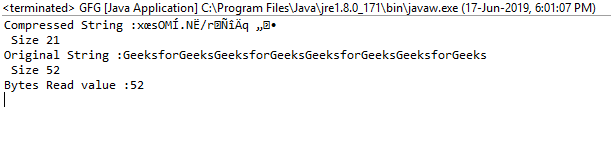# Deflater getBytesRead() function in Java with examples

The getBytesRead() function of the Deflater class in java.util.zip returns the total number of uncompressed bytes input provided till now.

Function Signature:

```public long getBytesRead()
```

Syntax:

```d.getBytesRead();
```

Parameter: The function requires no parameter

Return Type: The function returns a Long value which is the total number of uncompressed bytes input.

Exception: The function does not throw any exception

Example 1:

 `// Java program to describe the use ` `// of getBytesRead() function ` ` `  `import` `java.util.zip.*; ` `import` `java.io.UnsupportedEncodingException; ` ` `  `class` `GFG { ` `    ``public` `static` `void` `main(String args[]) ` `        ``throws` `UnsupportedEncodingException ` `    ``{ ` `        ``// deflater ` `        ``Deflater d = ``new` `Deflater(); ` ` `  `        ``// get the text ` `        ``String pattern = ``"GeeksforGeeks"``, text = ``""``; ` ` `  `        ``// generate the text ` `        ``for` `(``int` `i = ``0``; i < ``4``; i++) ` `            ``text += pattern; ` ` `  `        ``// set the input for deflator ` `        ``d.setInput(text.getBytes(``"UTF-8"``)); ` ` `  `        ``// finish ` `        ``d.finish(); ` ` `  `        ``// output bytes ` `        ``byte` `output[] = ``new` `byte``[``1024``]; ` ` `  `        ``// compress the data ` `        ``int` `size = d.deflate(output); ` ` `  `        ``// compressed String ` `        ``System.out.println(``"Compressed String :"` `                           ``+ ``new` `String(output) ` `                           ``+ ``"\n Size "` `+ size); ` ` `  `        ``// original String ` `        ``System.out.println(``"Original String :"` `                           ``+ text + ``"\n Size "` `                           ``+ text.length()); ` ` `  `        ``// get the total number of ` `        ``// uncompressed bytes input so far ` `        ``System.out.println(``"Bytes Read value :"` `                           ``+ d.getBytesRead()); ` ` `  `        ``// end ` `        ``d.end(); ` `    ``} ` `} `

Output:

```Compressed String :x?sOM?.N?/r???q??
Size 21
Original String :GeeksforGeeksGeeksforGeeksGeeksforGeeksGeeksforGeeks
Size 52
```Attention reader! Don’t stop learning now. Get hold of all the important Java and Collections concepts with the Fundamentals of Java and Java Collections Course at a student-friendly price and become industry ready.

My Personal Notes arrow_drop_upCheck out this Author's contributed articles.

If you like GeeksforGeeks and would like to contribute, you can also write an article using contribute.geeksforgeeks.org or mail your article to contribute@geeksforgeeks.org. See your article appearing on the GeeksforGeeks main page and help other Geeks.

Please Improve this article if you find anything incorrect by clicking on the "Improve Article" button below.

Article Tags :
Practice Tags :

Be the First to upvote.

Please write to us at contribute@geeksforgeeks.org to report any issue with the above content.